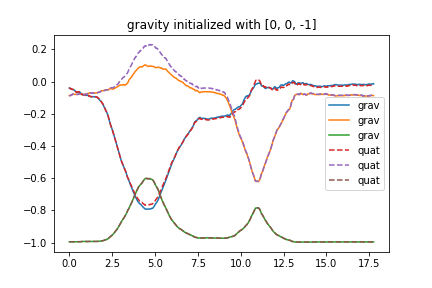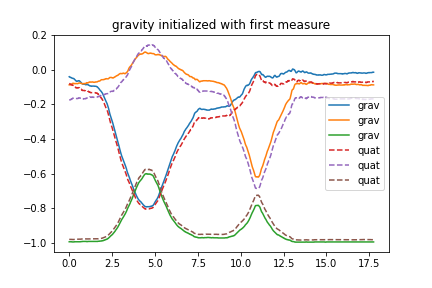# sign problem with gravity

I made a log with gentle rotations will recording gravity an quaternion.
Then I tried to check the consistency of both information. With matamotion R oriented as show in tutorial (https://mbientlab.com/tutorials/Orientation.html ) the z value is positive. I expected it to be negative.

Then I calculate the gravity at every time by two ways

• rotation of unit vertical downward with quaternion
•• rotation of initial gravity measurement with quaternion(to obtain right sign for x and y I needed to change sign of the z measured value
On both figures order is "x y z". grav are the measurements and quat are teh values computed with qauternions

So what is the reasaon for the sign convention ?